# Reflexive space

(diff) ← Older revision | Latest revision (diff) | Newer revision → (diff)
A Banach spacethat coincides under the canonical imbedding with its second dual(cf. Adjoint space). More precisely, let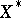be the space dual to, i.e. the set of all continuous linear functionals defined on. If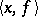is the value of the functional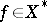on an element, then with a fixedandrunning through, the formula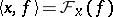defines a linear functional on, that is, an element of the space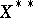. Let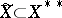be the set of such functionals. The correspondence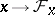is an isomorphism which does not change the norm:. If, then the spaceis called reflexive. The spaces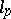and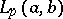,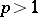, are reflexive, and the space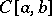is not reflexive.
A spaceis reflexive if and only if the spaceis reflexive. Another criteria of reflexivity of a Banach spaceis weak compactness (cf. Weak topology) of the unit ball of this space.